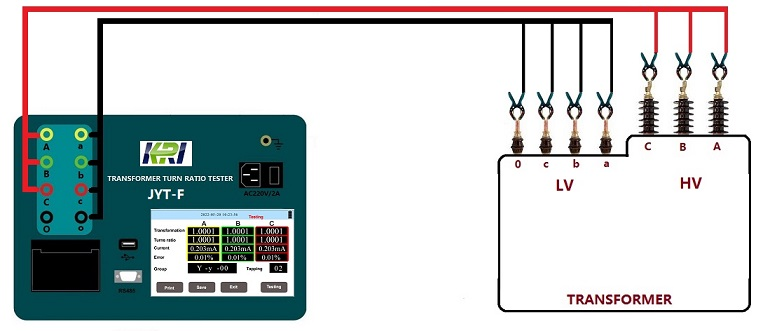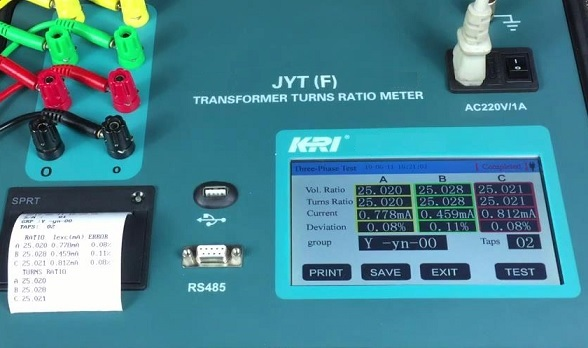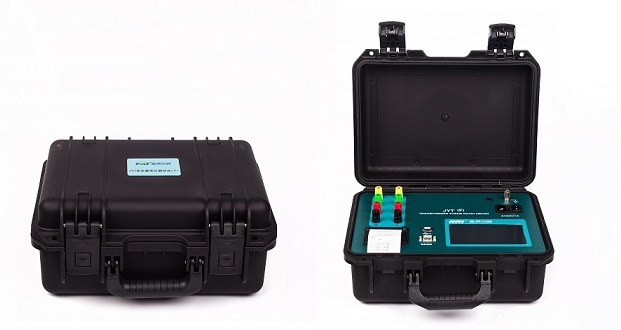Industry News

# Why is the ratio and turn ratio in the transformer test different?

Under normal circumstances, everyone thinks that the turn ratio of the transformer is the transformer ratio, or the ratio is the turn ratio, but is the actual situation like this? Let us start with the concept of transformer ratio and the turn ratio.

The transformer turns ratio is the ratio of the number of primary turns (that is, the number of turns of the coil) to the number of secondary turns. The turns ratio is proportional to the voltage ratio. For example, the ratio of 220 volts to 10 volts is 220:10, and the turns ratio should also be 220:10. For example, the primary is 2200 laps, and the secondary should be 100 laps. The multi-turn ratio is the number of turns and the primary corresponding to the secondary output.

The transformer ratio is the ratio of the voltage or current between the primary winding and the secondary winding. For example, the input voltage of the primary winding of the transformer is 110kV, and the output voltage of the secondary winding is 11kV, then the transformer ratio is 10

According to the formula, the theoretical ratio is the turns ratio. However, in practice, the turns ratio is determined by the ratio, the secondary required power (copper loss), and the magnetic flux of the core silicon steel sheet. Because the voltage transformer has the core loss and the excitation current, in order to ensure the accuracy, it needs to be compensated, that is, the reduction compensation, so the ratio is generally greater than the turns ratio. Assuming that a transformer (voltage transformer is a transformer) requires a ratio of 10/1, then the turns ratio cannot be 10/1 (eg primary 100 laps, secondary 10 laps). Maybe 9/1, such as the primary 90 laps, the secondary 10 laps; or the primary 100 laps, the secondary 11 laps, which is 9/1, because the purpose is to make the secondary actual voltage slightly higher than the theoretical value. . In this sense, 10/1 is greater than 9/1, so the ratio is greater than the turns ratio.

Kingrun’s transformer turn ratio tester(JYT-F) is a professional testing instrument that can test both turn ratio and ratio of transformer, which is suitable for transformer service, substation maintenance, power transformer /distribution transformer maintenance field,  Kingrun series tun ratio tester such as JYT-F and JYT-A tester has reliable capability and accurate testing result selected by SIEMENS and ABB transformer manufacture, for more information, please log on Kingrun website: www.kritester.com

JYT-F Technology Specification:

 Type JYT-F Figures on display 5 Five figure Output voltage AC160V AV10V Auto (DC battery) Test range 0.9 -10000 0.9 - 500 0.9 - 5000 Accuracy <500 0.1% ±2 Character ≤150 0.1% ±2 Character ≤1000 0.1% ±2 Character 500-2000 0.2% ±2 Character 150-       500 0.3% ±2 Character 1000-5000 0.3% ±2 Character >2000 0.3% ±2Character Minimum resolution 0.0001 Rechargeable lithium battery Power supply in operation AC220V±10%,50Hz±1Hz Test storied data 50 Groups Temperature in operation -20~40℃ Relative humidity ≤80%RH,  No forming dew Volume / Weight Length 395mm Width 240mm Height 250mm / 6 kgs